# RTM Deep Learning Style

In my quest to better understand deep learning, I have attempted to replicate some basic models I am familiar with in criminology, just typical OLS and the more complicated group based trajectory models. Another example I will illustrate is doing a variant of Risk Terrain Modeling.

The typical way RTM is done is:

Data Prep Part:

1. create a set of independent variables for crime generators (e.g. bars, subway stops, liquor stores, etc.) that are either the distance to the nearest or the kernel density estimate
2. Turn these continuous estimates into dummy variables, e.g. if within 100 meters = 1, else = 0. For kernel density they typically z-score and if a z-score > 2 the dummy variable equals 1.
3. Do 2 for varying distance/bandwidth selections, e.g. 100 meters, 200 meters, etc. So you end up with a collection of distance variables, e.g. Bars_100, Bars_200, Bars_400, etc.

Modeling Part

1. Fit a Lasso regression predicting your crime outcome constraining all of the variables to be positive. (So RTM will never say a crime generator has a negative effect.)
2. For the variables that passed this Lasso stage, then do a variable selection routine. So instead of the final model having Bars_100 and Bars_400, it will only choose one of those variables.

For the modeling part, we can replicate various parts of these in a deep learning environment. For the constrain the coefficients to be positive, when you see folks refer to a “RelU” or the rectified linear unit function, all this means is that the coefficients are constrained to be positive. For the variable selection part, I needed to hack my own – it ends up being a combo of a custom dropout scheme and then pruning in deep learning lingo.

Although RTM is typically done on raster grid cells for the spatial unit of analysis, this is not a requirement. You can do all these steps on vector (e.g. street segments) or other areal spatial units of analysis.

Here I illustrate using street units (intersections and street segments) from DC. The crime generator data I take from my dissertation (and I have a few pubs in Crime & Delinquency based on that work). The crime data I illustrate using 2011 violent Part 1 UCR (homicide, agg assault, robbery, no rape in the public data).

The crime dataset is over time, and I describe in an analysis (with Billy Zakrzewski) on examining pre/post crime around DC medical marijuana dispensaries.

The data and code to replicate can be downloaded here. It is python, and for the deep learning model I used pytorch.

# RTM Example in Python

So I will walk through briefly my second script, `01_DeepLearningRTM.py`. The first script, `00_DataPrep.py`, does the data prep, so this data file already has the crime generator variables prepared in the manner RTM typically creates them. (The `rtm_dl_funcs.py` has the functions to do the feature extraction and create the deep learning model, to do distance/density in sci-kit is very slick, only a few lines of code.)

So first I just define the libraries I will be using, and import my custom rtm functions I created.

``````######################################################
import numpy as np
import pandas as pd
import torch
device = torch.device("cuda:0")
import os
import sys

my_dir = r'C:\Users\andre\OneDrive\Desktop\RTM_DeepLearning'
os.chdir(my_dir)
sys.path.append(my_dir)
import rtm_dl_funcs
######################################################``````

The next set of code grabs the crime data, and then defines my variable sets. I have plenty more crime generator data from my dissertation, but to make it easier on myself I just focus on distance to metro entrances, the density of 311 calls (a measure of disorder), and the distance and density of alcohol outlets (this includes bars/liquor stores/gas stations that sell beer, etc.).

Among these variable sets, the final selected model will only choose one within each set. But I have also included the ability for the model to incorporate other variables that will just enter in no-matter what (and are not constrained to be positive). This is mostly to incorporate an intercept into the regression equation, but here I also include the percent of sidewalk encompassing one of my street units (based on the Voronoi tessellation), and a dummy variable for whether the street unit is an intersection. (I also planned on included the area of the tessalation, but it ended up being an explosive effect, my dissertation shows its effect is highly non-linear, so didn’t want to worry about splines here for simplicity.)

``````######################################################
#Get the Prepped Data
crime_data = pd.read_csv('Prepped_Crime.csv')

#Variable sets for each
db = [50, 100, 200, 300, 400, 500, 600, 700, 800]
metro_set = ['met_dis_' + str(i) for i in db]
alc_set = ['alc_dis_' + str(i) for i in db]
alc_set += ['alc_kde_' + str(i) for i in db]
c311_set = ['c31_kde_' + str(i) for i in db]

#Creating a few other generic variables
crime_data['PercSidewalk'] = crime_data['SidewalkArea'] / crime_data['AreaMinWat']
crime_data['Const'] = 1
const_li = ['Const','Intersection','PercSidewalk']
full_set = const_li + alc_set + metro_set + c311_set
######################################################``````

The next set of code turns my data into a set of torch tensors, then I grab the size of my independent variable sets, which I will end up needing when initializing my pytorch model.

Then I set the seed (to be able to reproduce the results), create the model, and set the loss function and optimizer. I use a Poisson loss function (will need to figure out negative binomial another day).

``````######################################################
#Now creating the torch tensors
x_ten = torch.tensor(crime_data[full_set].to_numpy(), dtype=float)
y_ten = torch.tensor(crime_data['Viol_2011'].to_numpy(), dtype=float)
out_ten = torch.tensor(crime_data['Viol_2012'].to_numpy(), dtype=float)

#These I need to initialize the deep learning model
gen_lens = [len(alc_set), len(metro_set), len(c311_set)]

#Creating the model
torch.manual_seed(10)

model = rtm_dl_funcs.RTM_torch(const=len(const_li),
gen_list=gen_lens)
criterion = torch.nn.PoissonNLLLoss(log_input=True, reduction='mean')
optimizer = torch.optim.Adam(model.parameters(), lr=0.001) #1e-4
print( model )
######################################################``````

If you look at the printed out model, it gives a nice summary of the different layers. We have our one layer for the fixed coefficients, and another three sets for our alcohol outlets, 311 calls, and metro entrances. We then have a final cancel layer. The idea behind the final cancel layer is that the variable selection routine in RTM can still end up not selecting any variables for a set. I ended up not using it here though, as it was too aggressive in this example. (So will need to tinker with that some more!)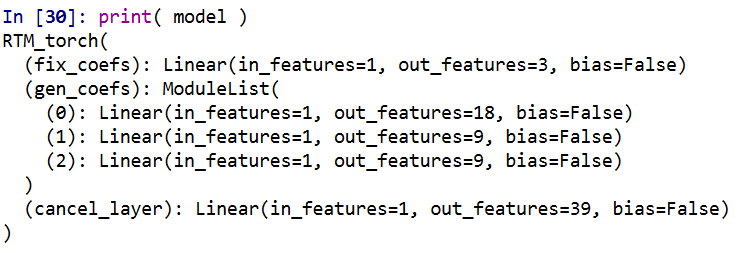The variable selection routine is very volatile – so if you have very correlated inputs, you can essentially swap one for the other and get near equivalent predictions. I often see folks who do RTM analyses say something along the lines of, “OK this RTM selected A, and this RTM selected B, so they are different effects in these two samples” (sometimes pre/post, other times comparing different areas, and other times different crime outcomes). I think this is probably wrong though to make that inference, as there is quite a bit of noise in the variable selection process (and the variable selection process itself precludes making inferences on the coefficients themselves).

My deep learning example inherited the same problems. So if you change the initialized weights, it may end up selecting totally different inputs in the end. To get the variable selection routine to at least select the same crime generator variables in my tests, I do a burn in period in which I implement a random dropout scheme. So instead of the typical dropout, for every forward pass it does a random dropout to only select one variable randomly out of each crime generator set. After that converges, I then use a pruning layer to only pick the coefficient that has the largest effect, and again do a large set of iterations to make sure the results converged. So different means but same ends to the typical RTM steps 4 and 5 above. I also have like I said a ReLU transformation after each layer, so the crime generator variables are always positive, any negative effects will be pruned out.

One thing that is nice about deep learning is that it can be quite fast. Here each of these 10,000 iteration sets take less than a minute on my desktop with a GPU. (I’ve been prototyping models with more parameters and more observations at work on my laptop with just a CPU that only take like 10 to 20 minutes).

``````######################################################
#Burn in part, random dropout
for t in range(10000):
#Forward pass
y_pred = model(x=x_ten)
#Loss
loss_insample = criterion(y_pred, y_ten)
optimizer.zero_grad()
loss_insample.backward(retain_graph=True)
optimizer.step()
if t % 1000 == 0:
print(f'loss: {loss_insample.item()}' )

#Switching to pruning all but the largest effects
model.l1_prune()

for t in range(10000):
#Forward pass
y_pred = model(x=x_ten, mask_type=None, cancel=False)
#Loss
loss_insample = criterion(y_pred, y_ten)
optimizer.zero_grad()
loss_insample.backward(retain_graph=True)
optimizer.step()
if t % 1000 == 0:
print(f'loss: {loss_insample.item()}' )

print( model.coef_df(nm_li=full_set, cancel=False) )
######################################################``````

And this prints out the results (as incident rate ratios), so you can see it selected 50 meters alcohol kernel density, 50 meters distance to the nearest metro station, and kernel density for 311 calls with an 800 meter bandwidth.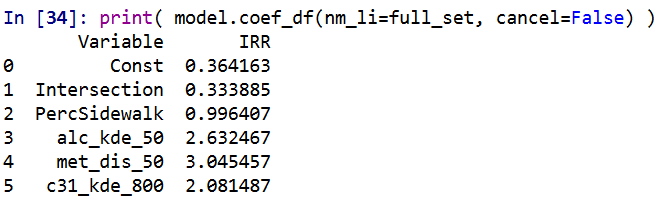I have in the code another example model when using a different seed. So testing out on around 5 different seeds it always selected these same distance/density variables, but the coefficients are slightly different each time. Here is an example from setting the seed to 12.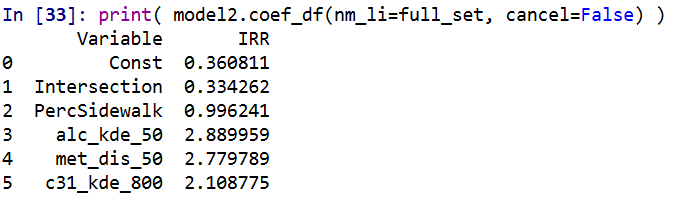These models are nothing to brag about, using the typical z-score the predictions and set the threshold to above 2, the PAI is only around 3 (both for in-sample 2011 and out of sample 2012 is slightly lower). It is a tough prediction task – the mean number of violent crimes per street unit per year is only 0.3. Violent crime is fortunately very rare!

But with only three different risk variables, we can do a quick conjunctive analysis, and look at the areas of overlap.

``````######################################################
#Adding model 1 predictions back into the dataset
pred_mod1 = pd.Series(model(x=x_ten, mask_type=None, cancel=False).exp().detach().numpy())
crime_data['Pred_M1'] = pred_mod1

#Check out the areas of overlapping risk
mod1_coef = model.coef_df(nm_li=full_set, cancel=False)
risk_vars = list(set(mod1_coef['Variable']) - set(const_li))
conj_set = crime_data.groupby(risk_vars, as_index=False)['Const','Pred_M1','Viol_2012'].sum()
print(conj_set)
######################################################``````

In this table `Const` is the total number of street units selected, `Pred_M1` is the expected number of crimes via Model 1, and then I show how well it conforms to the predictions out of sample 2012. So you can see in the aggregate the predictions are not too far off. There only ends up being one street unit that overlaps for all three risk factors in the study area.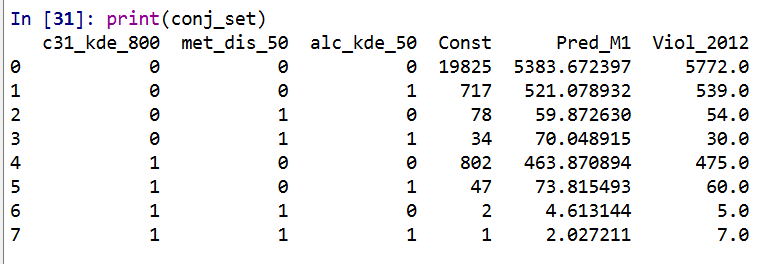I believe the predictions would be better if I included more crime generator variables. But ultimately the nature of how RTM works it trades off accuracy for simple models. Which is fair – it helps to ease the nature of how a police department (or some other entity) responds to the predictions.

But this trade off results in predictions that don’t fare as well compared with more complicated models. For example I show (with Wouter Steenbeek) that random forests do much better than RTM. To make those models more interpretable we did local decompositions for hot spots, so say this hot spot is 30% alcohol outlets, 20% nearby apartments, etc.

So there is no doubt more extensions for RTM you could do in a deep learning framework, but they will likely always result in more complicated and less interpretable models. Also here I don’t think this code will be better than the traditional RTM folks, the only major benefit of this code is it will run faster – minutes instead of overnight for most jobs.

# New preprint: Allocating police resources while limiting racial inequality

I have a new working paper out, Allocating police resources while limiting racial inequality. In this work I tackle the problem that a hot spots policing strategy likely exacerbates disproportionate minority contact (DMC). This is because of the pretty simple fact that hot spots of crime tend to be in disadvantaged/minority neighborhoods.

Here is a graph illustrating the problem. X axis is the proportion of minorities stopped by the police in 500 by 500 meter grid cells (NYPD data). Y axis is the number of violent crimes over along time period (12 years). So a typical hot spots strategy would choose the top N areas to target (here I do top 20). These are all very high proportion minority areas. So the inevitable extra police contact in those hot spots (in the form of either stops or arrests) will increase DMC.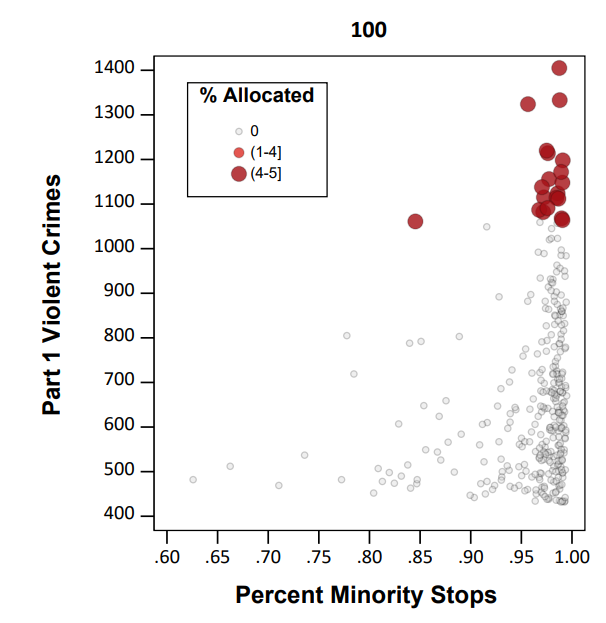I’d note that the majority of critiques of predictive policing focus on whether reported crime data is biased or not. I think that is a bit of a red herring though, you could use totally objective crime data (say swap out acoustic gun shot sensors with reported crime) and you still have the same problem.

The proportion of stops by the NYPD of minorities has consistently hovered around 90%, so doing a bunch of extra stuff in those hot spots will increase DMC, as those 20 hot spots tend to have 95%+ stops of minorities (with the exception of one location). Also note this 90% has not changed even with the dramatic decrease in stops overall by the NYPD.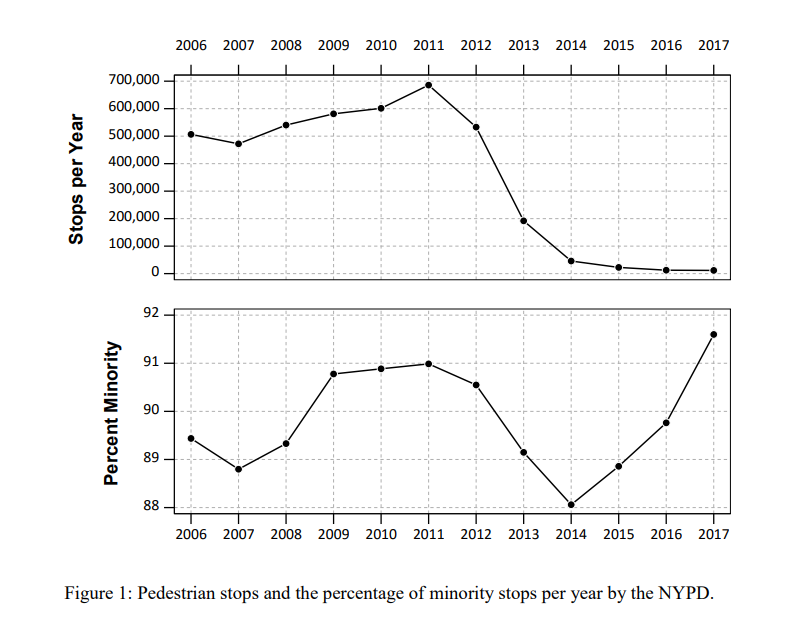So to illustrate my suggested solution here is a simple example. Consider you have a hot spot with predicted 30 crimes vs a hot spot with predicted 28 crimes. Also imagine that the 30 crime hot spot results in around 90% stops of minorities, whereas the 28 crime hot spot only results in around 50% stops of minorities. If you agree reducing DMC is a reasonable goal for the police in-and-of-itself, you may say choosing the 28 crime area is a good idea, even though it is a less efficient choice than the 30 crime hot spot.

I show in the paper how to codify this trade-off into a linear program that says choose X hot spots, but has a constraint based on the expected number of minorities likely to be stopped. Here is an example graph that shows it doesn’t always choose the highest crime areas to meet that racial equity constraint.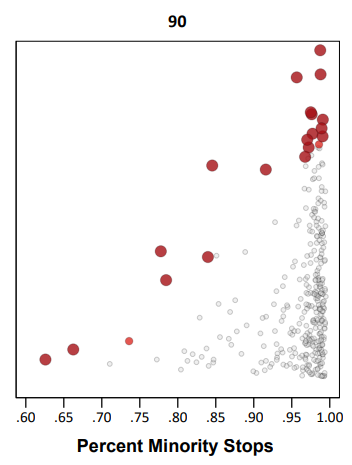This results in a trade-off of efficiency though. Going back to the original hypothetical, trading off a 28 crime vs 30 crime area is not a big deal. But if the trade off was 3 crimes vs 30 that is a bigger deal. In this example I show that getting to 80% stops of minorities (NYC is around 70% minorities) results in hot spots with around 55% of the crime compared to the no constraint hot spots. So in the hypothetical it would go from 30 crimes to 17 crimes.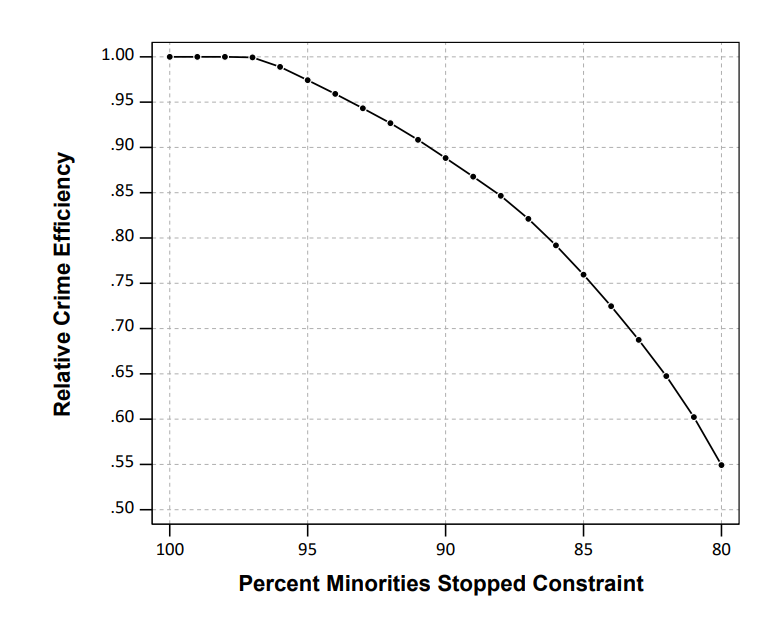There won’t be a uniform formula to calculate the expected decrease in efficiency, but I think getting to perfect equality with the residential pop. will typically result in similar large decreases in many scenarios. A recent paper by George Mohler and company showed similar fairly steep declines. (That uses a totally different method, but I think will be pretty similar outputs in practice — can tune the penalty factor in a similar way to changing the linear program constraint I think.)

So basically the trade-off to get perfect equity will be steep, but I think the best case scenario is that a PD can say "this predictive policing strategy will not make current levels of DMC worse" by applying this algorithm on-top-of your predictive policing forecasts.

I will be presenting this work at ASC, so stop on by! Feedback always appreciated.

# New preprint: The accuracy of the violent offender identification directive (VOID) tool to predict future gun violence

I have a new preprint out, The accuracy of the violent offender identification directive (VOID) tool to predict future gun violence. This is work with Rob Worden and Jasmine Silver from our time at the Finn Institute. Below is the abstract:

We evaluate the Violent Offender Identification Directive (VOID) tool, a risk assessment instrument implemented within a police department to prospectively identify offenders likely to be involved with future gun violence. The tool uses a variety of static measures of prior criminal history that are readily available in police records management systems. The VOID tool is assessed for predictive accuracy by taking a historical sample and calculating scores for over 200,000 individuals known to the police at the end of 2012, and predicting 103 individuals involved with gun violence (either as a shooter or a victim) during 2013. Despite weights for the instrument being determined in an ad-hoc manner by crime analysts, the VOID tool does very well in predicting involvement with gun violence compared to an optimized logistic regression and generalized boosted models. We discuss theoretical reasons why such ad-hoc instruments are likely to perform well in identifying chronic offenders for all police departments.

There were just slightly over 100 violent gun offenders we were trying to pick out of over 200,000. The VOID tool did really well! Here is a graph comparing how many of those offenders VOID captured compared to a generalized boosted model (GBM), and two different logistic regression equations.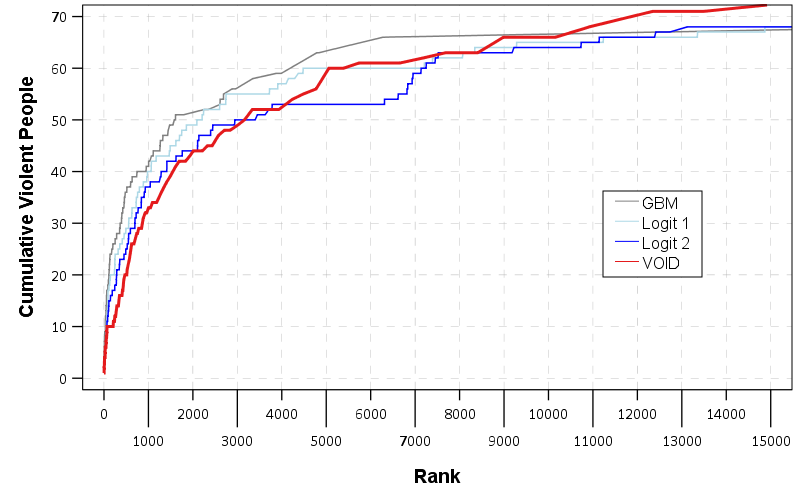I have some of my thoughts in this article as to why a simple tool does just as well as more complicated regression and machine learning techniques, which is a common finding in recidivism studies as well. My elevator pitch for why that is is because most offenders are generalists, and for example you can basically swap prior arrests for robbery with prior arrests for motor vehicle theft — they both provide essentially the same signal for future potential criminality. See also discussion of this on Dan Simpson’s post on the Stat Modeling, Causal Inference and Social Science blog, which in turn makes me think the idea behind simple models can be readily applied to many decision points in the criminal justice field.

The simple takeaway from this for crime analysts making chronic offender lists is that don’t let the perfect be the enemy of the good. Analysts can likely create an ad-hoc weighting to prioritize chronic offenders and it will do quite well compared to fancier models.

I will be presenting this work at the ACJS conference in New Orleans on Saturday 2/17/18. It is a great session, with YongJei Lee, Jerry Ratcliffe, Bryanna Fox, and Stacy Sechrist (see session 384 in the ACJS program), so stop on by. If you want to catch up with me in New Orleans just send me an email. And as always if you have feedback on the draft I am all ears.

# How wide to make the net in actuarial tools? (false positives versus false negatives)

An interesting debate/question came up in my work recently. I conducted an analysis of a violence risk assessment tool for a police department. Currently the PD takes around the top 1,000 scores of this tool, and then uses further intelligence and clinical judgements to place a small number of people on a chronic offender list (who are then subject to further interventions). My assessment of the predictive validity when examining ROC curves suggested the tool does a pretty good job discriminating violent people up to around the top 6,000 individuals and after that flattens out. In a sample of over 200,000, the top 1000 scores correctly classified 30 of the 100 violent cases, and the top 6000 classified 60.

So the question came up should we recommend that the analysts widen the net to the top 6,000 scores, instead of only examining the top 1,000 scores? There are of course costs and limitations of what the analysts can do. It may simply be infeasible for the analysts to review 6,000 people. But how do you set the limit? Should the clinical assessments be focused on even fewer individuals than 1,000?

We can make some estimates of where the line should be drawn by setting weights for the cost of a false positive versus a false negative. Implicit in the whole exercise of predicting violence in a small set of people is that false negatives (failing to predict someone will be violent when they are) greatly outweigh a false positive (predicting someone will be violent but they are not). The nature of the task dictates that you will always need to have quite a few false positives to classify even a few true positives, and no matter what you do there will only be a small number of false negatives.

Abstractly, you can place a value on the cost of failing to predict violence, and a cost on the analysts time to evaluate cases. In this situation we want to know whether the costs of widening the net to 6,000 individuals are less than the costs of only examining the top 1,000 individuals. Here I will show we don’t even need to know what the exact cost of a false positive or a false negative is, only the relative costs, to make an estimate about whether the net should be cast wider.

The set up is that if we only take the top 1,000 scores, it will capture 30 out of the 100 violent cases. So there will be (100 – 30) false negatives, and (1000 – 30) false positives. If we increase the scores to evaluate the top 6,000, it will capture 60 out the 100 violent cases, but then we will have (6000 – 60) false positives. I can not assign a specific number to the cost of a false negative and a false positive. So we can write these cost equations as:

``````1) (100 - 30)*FN + (1000 - 30)*FP = Cost Low
2) (100 - 60)*FN + (6000 - 60)*FP = Cost High``````

Even though we do not know the exact cost of a false negative, we can talk about relative costs, e.g. `1 false negative = 1000*false positives`. There are too many unknowns here, so I am going to set `FP = 1`. This makes the numbers relative, not absolute. So with this constraint the reduced equations can be written as:

``````1) 70*FN +  970 = Cost Low
2) 40*FN + 5940 = Cost High``````

So we want to know the ratio at which there is a net benefit over including the top 6,000 scores versus only the top 1,000. So this means that `Cost High < Cost Low`. To figure out this point, we can subtract equation 2 from equation 1:

``3) (70 - 40)*FN - 4970 = Cost Low - Cost High``

If we set this equation to zero and solve for FN we can find the point where these two equations are equal:

``````30*FN - 4970 = 0
30*FN = 4970
FN = 4970/30 = 165 + 2/3``````

If the value of a false negative is more than 166 times the value of a false positive, `Cost Low - Cost High` will be positive, and so the false negatives are more costly to society relative to the analysts time spent. It is still hard to make guesses as to whether the cost of violence to society is 166 times more costly than the analysts time, but that is at least one number to wrap your head around. In a more concrete example, such as granting parole or continuing to be incarcerated, given how expensive prison is net widening (with these example numbers) would probably not be worth it. But here it is a bit more fuzzy especially because the analysts time is relatively inexpensive. (You also have to guess how well you can intervene, in the prison example incarceration essentially reduces the probability of committing violence to zero, whereas police interventions can not hope to be that successful.)

As long as you assume that the classification rate is linear within this range of scores, the same argument holds for net widening any number. But in reality there are diminishing returns the more scores you examine (and 6,000 is basically where the returns are near zero). If you conduct the same exercise between classifying zero and the top 1,000, the rate of the cost of a false negative to a false positive needs be `32+1/3` to justify evaluating the top 1,000 scores. If you actually had an estimate of the ratio of the cost of false positives to false negatives you could then figure out exactly how wide to make the net. But if you think the ratio is well above 166, you have plenty of reason to widen the net to the larger value.

# ROC and Precision-Recall curves in SPSS

Recently I was tasked with evaluating a tool used to predict violence. I initially created some code to plot ROC curves in SPSS for multiple classifiers, but then discovered that the `ROC` command did everything I wanted. Some recommend precision-recall curves in place of ROC curves, especially when the positive class is rare. This fit my situation (a few more than 100 positive cases in a dataset of 1/2 million) and it was pretty simple to adapt the code to return the precision. I will not go into the details of the curves (I am really a neophyte at this prediction stuff), but here are a few resources I found useful:

The macro is named `!Roc` and it takes three parameters:

• `Class` – the numeric classifier (where higher equals a greater probability of being predicted)
• `Target` – the outcome you are trying to predict. Positive cases need to equal 1 and negative cases 0
• `Suf` – this the the suffix on the variables returned. The procedure returns “Sens[Suf]”, “Spec[Suf]” and “Prec[Suf]” (which are the sensitivity, specificity, and precision respectively).

So here is a brief made up example using the macro to draw ROC and precision and recall curves (entire syntax including the macro can be found here). So first lets make some fake data and classifiers. Here `Out` is the target being predicted, and I have two classifiers, `X` and `R`. `R` is intentionally made to be basically random. The last two lines show an example of calling the macro.

``````SET SEED 10.
INPUT PROGRAM.
LOOP #i = 20 TO 70.
COMPUTE X = #i + RV.UNIFORM(-10,10).
COMPUTE R = RV.NORMAL(45,10).
COMPUTE Out = RV.BERNOULLI(#i/100).
END CASE.
END LOOP.
END FILE.
END INPUT PROGRAM.
DATASET NAME RocTest.
DATASET ACTIVATE RocTest.
EXECUTE.

!Roc Class = X Target = Out Suf = "_X".
!Roc Class = R Target = Out Suf = "_R".``````

Now we can make an ROC curve plot with this information. Here I use inline `TRANS` statements to calculate 1 minus the specificity. I also use a blending trick in GPL to make the beginning of the lines connect at (0,0) and the end at (1,1).

``````*Now make a plot with both classifiers on it.
GGRAPH
/GRAPHDATASET NAME="graphdataset" VARIABLES=Spec_X Sens_X Spec_R Sens_R
/GRAPHSPEC SOURCE=INLINE.
BEGIN GPL
PAGE: begin(scale(770px,600px))
SOURCE: s=userSource(id("graphdataset"))
DATA: Spec_X=col(source(s), name("Spec_X"))
DATA: Sens_X=col(source(s), name("Sens_X"))
DATA: Spec_R=col(source(s), name("Spec_R"))
DATA: Sens_R=col(source(s), name("Sens_R"))
TRANS: o = eval(0)
TRANS: e = eval(1)
TRANS: SpecM_X = eval(1 - Spec_X)
TRANS: SpecM_R = eval(1 - Spec_R)
COORD: rect(dim(1,2), sameRatio())
GUIDE: axis(dim(1), label("1 - Specificity"), delta(0.1))
GUIDE: axis(dim(2), label("Sensitivity"), delta(0.1))
GUIDE: text.title(label("ROC Curve"))
SCALE: linear(dim(1), min(0), max(1))
SCALE: linear(dim(2), min(0), max(1))
ELEMENT: edge(position((o*o)+(e*e)), color(color.lightgrey))
ELEMENT: line(position(smooth.step.right((o*o)+(SpecM_R*Sens_R)+(e*e))), color("R"))
ELEMENT: line(position(smooth.step.right((o*o)+(SpecM_X*Sens_X)+(e*e))), color("X"))
PAGE: end()
END GPL.``````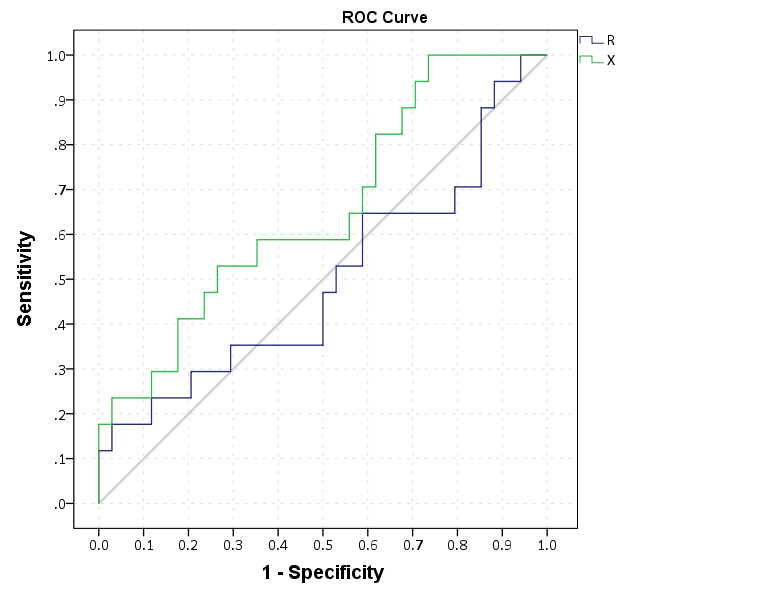This just replicates the native SPSS `ROC` command though, and that command returns other useful information as well (such as the actual area under the curve). We can see though that my calculations of the curve are correct.

``````*Compare to SPSS's ROC command.
ROC R X BY Out (1)
/PLOT CURVE(REFERENCE)
/PRINT SE COORDINATES.``````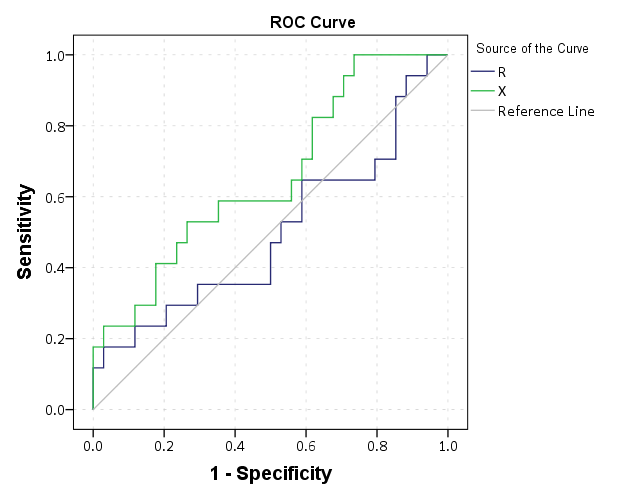To make a precision-recall graph we need to use the `path` element and sort the data in a particular way. (SPSS’s `line` element works basically the opposite of the way we need it to produce the correct sawtooth pattern.) The blending trick does not work with this graph, but it is immaterial in interpreting the graph.

``````*Now make precision recall curves.
*To make these plots, need to reshape and sort correctly, so the path follows correctly.
VARSTOCASES
/MAKE Sens FROM Sens_R Sens_X
/MAKE Prec FROM Prec_R Prec_X
/MAKE Spec FROM Spec_R Spec_X
/INDEX Type.
VALUE LABELS Type
1 'R'
2 'X'.
SORT CASES BY Sens (A) Prec (D).
GGRAPH
/GRAPHDATASET NAME="graphdataset" VARIABLES=Sens Prec Type
/GRAPHSPEC SOURCE=INLINE.
BEGIN GPL
PAGE: begin(scale(770px,600px))
SOURCE: s=userSource(id("graphdataset"))
DATA: Sens=col(source(s), name("Sens"))
DATA: Prec=col(source(s), name("Prec"))
DATA: Type=col(source(s), name("Type"), unit.category())
COORD: rect(dim(1,2), sameRatio())
GUIDE: axis(dim(1), label("Recall"), delta(0.1))
GUIDE: axis(dim(2), label("Precision"), delta(0.1))
GUIDE: text.title(label("Precision-Recall Curve"))
SCALE: linear(dim(1), min(0), max(1))
SCALE: linear(dim(2), min(0), max(1))
ELEMENT: path(position(Sens*Prec), color(Type))
PAGE: end()
END GPL.
*The sawtooth is typical.``````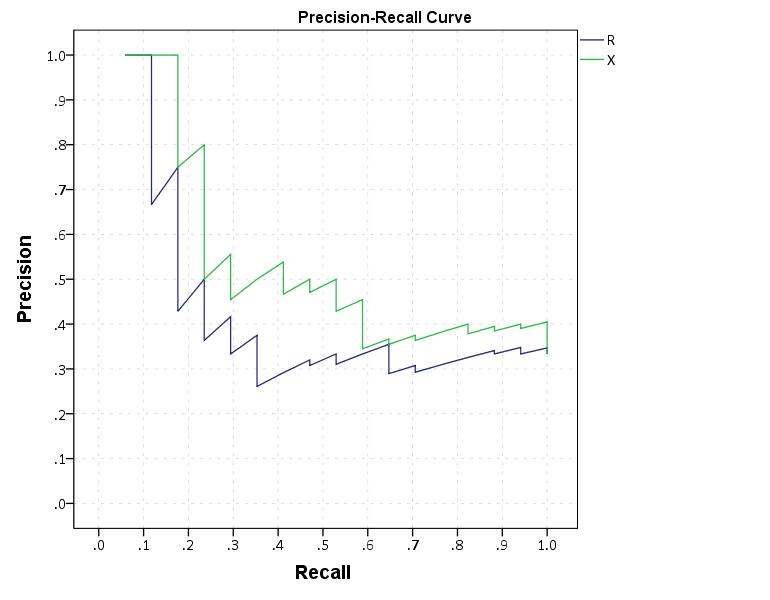These curves both show that `X` is the clear winner. In my use application the ROC curves are basically superimposed, but there is more separation in the precision-recall graph. Being very generic, most of the action in the ROC curve is at the leftmost area of the graph (with only a few positive cases), but the PR curve is better at identifying how wide you have to cast the net to find the few positive cases. In a nut-shell, you have to be willing to live with many false positives to be able to predict just the few positive cases.

I would be interested to hear other analysts perspective. Predicting violence is a popular topic in criminology, with models of varying complexity. But what I’m finding so far in this particular evaluation is basically that there are set of low hanging fruit of chronic offenders that score high no matter how much you crunch the numbers (around 60% of the people who committed serious violence in a particular year in my sample), and then a set of individuals with basically no prior history (around 20% in my sample). So basically ad-hoc scores are doing about as well predicting violence as more complicated machine learning models (even machine learning models fit on the same data).

• ## Follow Blog via Email

Enter your email address to follow this blog and receive notifications of new posts by email.

Join 257 other followers